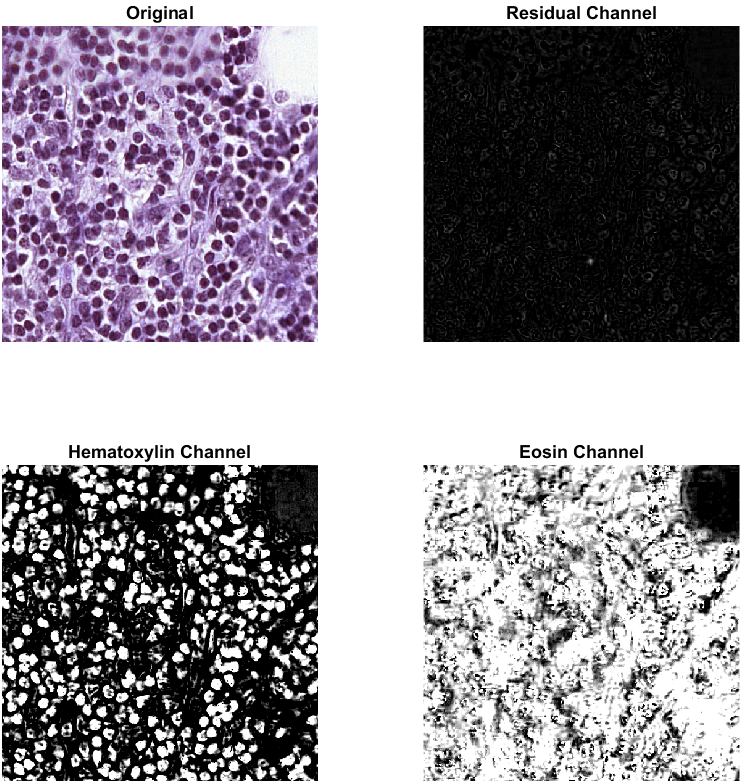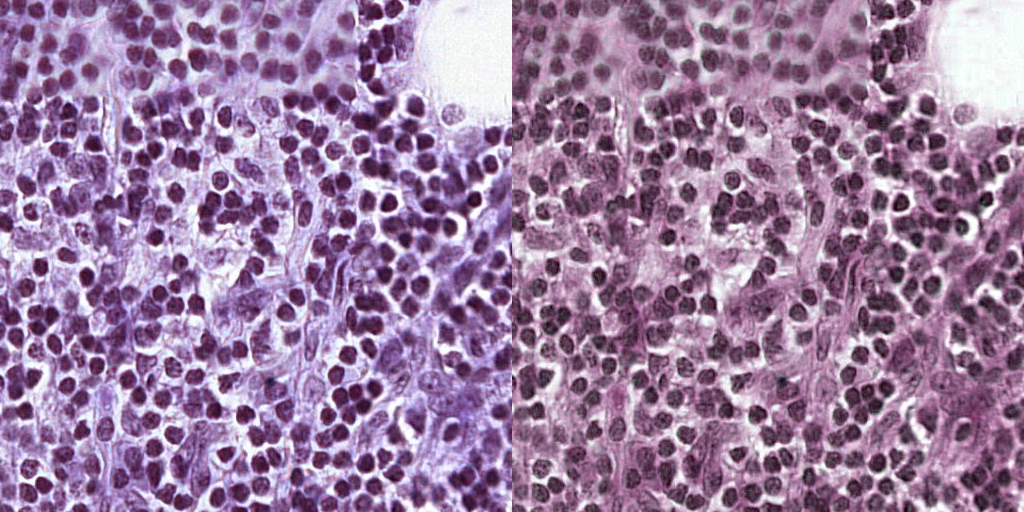Warning

## Lab 02 : Microscopy

Topics:

• Exercises on optics, microscopy etc.
• Stain normalization for histo-pathology images

### HW02 Assignment

Homework HW02 has two parts, detailed description follows:

### (A) Excercises

Important equations

• konvenční zraková vzdálenost optical distance $d = 250 mm$
• zvětšení magnification $Z = \frac{y'}{y} = -\frac{a'}{a}$
• zobrazovací rovnice spojné čočky lense maker's formula $\frac{1}{a} + \frac{1}{a'} = \frac{1}{f}$ with $a, a'$ the object's and
• zvětšení mikroskopu magnification of a microscope

$$Z = \frac{\Delta}{f_{ob}} \frac{d}{f_{oc}}$$

• Abbé-Rayleigh criterion $d = 1.22 \cdot \frac{\lambda}{2 \cdot NA}$

#### Lens

Let us consider a thin convex lens (spojná čočka) with focal point distance of 10 cm. Find the magnification for a red object of height 6 cm, placed in the distance of 12 cm in front of the imaging plane.

Solution Given: $f=0.1~m, y=0.06~m, a=0.12~m$ Magnification is the ratio of the size of the displayed object to the size of the original object $Z = \frac{y'}{y} = \frac{a'}{a}$ and the imaging equation gives us $a' = \frac{f \cdot a}{a - f}$ and finally, after substituting $a'$, we get $$Z = \frac{f}{a - f} = \frac{0.1}{0.12 - 0.1} = 5$$

#### Microscope I

Consider a simple microscope formed by two thin convex lenses with objective focal length 5 mm and diameter of 1 cm, tube optical length 100 mm and eyepiece focal distance of 25 mm. Draw a schematic picture of the microscope and compute its magnification.

Solution Given is $f_\text{ob} = 0.005$, $f_\text{oc} = 0.025$, tube length $\Delta = 0.1 m$ and conventional optical distance $d=0.25$m. By simple insertion into the magnification equation, we get

$$Z = \frac{\Delta}{f_{\text{ob}}} \cdot \frac{d}{f_{\text{oc}}} = \frac{0.1}{0.25} \frac{0.005}{0.025} = 200.$$

#### (HW) Microscope II

Consider a simple microscope with an objective magnification of 40, eyepiece magnification of 10 and tube length of 12 cm. Compute the magnification as well as the focal lengths of the objective and the eyepiece.

#### Microscope resolution

Let us consider a microscope with eyepiece numerical aperture (NA) of 0.4, using illumination by light of wavelength 650 nm. Compute the maximal resolution (minimal distance between distinguishable points) we can achieve in this setting.

Solution From Abbe-Rayleigh criterion: $$d = 1.22 \frac{\lambda}{2 NA} = 1.22 \cdot \frac{6.5 \cdot 10^{-7}}{2 \cdot 0.4} = 990 ~n \text{m} = 1 ~\mu \text{m} \,$$

#### (HW) Microscope resolution

Let us consider a lens $L$ with a half-cone angle $\theta=30^\circ$ in immersion oil ($n = 1.33$) and normal light illumination ($\lambda$ = 650 nm). Which light (wavelength and color) do we need to use to get the same resolution capabilities when using air ($n=1$) as a diffraction medium?

### (B) Stain normalization

Histopathology images are microscopy images of small tissue samples (cuts or slices). Since cells are usually colorless, we use the technique of staining to make important structures visible in the microscope. For this, the tissue probe is put into a solution with two dyes, most commonly Hematoxylin and Eosin. They are both colorless chemical compounds with different properties

• Hematoxylin binds to basophilic structures, such as DNA, RNA and colors them dark blue or violet
• Eosin, on the contrary, binds to acidophilic structures like protein chains and colors them pink

Different dye concentration leads to differently colored images. This effect can be further emphasized by different lighting conditions during image acquisition. To apply image segmentation or classification techniques, we want the color variability to be minimal. To achieve this, we usually apply a stain normalization technique.

Color (de-convolution) According to the Beer-Lambert law, there is an exponential relationship between the intensity of the transmitted light $I_\lambda(\mathbf{x})$ at wavelength $\lambda$ in a 2D point $\mathbf{x}$ and the amount of absorbed dye $n(\mathbf{x})$, given by the following equation

$$I_\lambda(x) = I^0_\lambda(x) {\rm e}^{-m_\lambda n(x)}$$

where $I^0_\lambda(x)$ is the intensity of the incident light and $m_\lambda$ is the attenuation coefficient of the stain. Let us define the optical density $d_\lambda(\mathbf{x}) = \log( \frac{I^0_\lambda(\mathbf{x})}{I_\lambda(\mathbf{x})})$, leading to $d_\lambda=m_\lambda n(\mathbf{x})$. We now assemble the individual $d_\lambda$ values into a 3D vector, corresponding to standard RGB colors:

$$\mathbf{d}=(d_R,d_G,d_B)=M \mathbf{n}(\mathbf{x})$$

where $M$ is a so-called color mixing or color convolution matrix. The vector $\mathbf{n}(x)$ also has three components - two representing the hematoxylin and eosin concentrations and the third representing the residual attenuation - impurities and model imperfections. The remaining step is to concatenate vectors $\mathbf{d}(\mathbf{x})$ and $\mathbf{n}(\mathbf{x})$ from all pixels in the image into $3 \times P$ matrices $\mathbf{D}$ and $\mathbf{N}$, where $P$ is the number of pixels.

$$\mathbf{D} = \mathbf{M} \mathbf{N}$$

The key tasks are the estimation of the mixing matrix $\mathbf{M}$ and reconstruction of the stain density image $\mathbf{N}$ from the observed optical density image $\mathbf{D}$ and thus identifying the concentration of the dyes. This is called color or stain deconvolution. Then it is possible to create images with an arbitrary but identical appearance by applying the same color mixing matrix $\mathbf{M}$ to all images. The resulting images are called stain normalized.

Processing hints

We start with an image $I$, where each pixel $I(x) = (r, g, b)$ has the color information in RGB color space. We then compute the optical density image $D = -\log{\frac{I+1}{I_0}}$ with the maximal intensity $I_0 = 255$ in our case (the +1 is to avoid zero within the logarithm). With a given color matrix $M_A$ we get the stains $N(x)$ by solving $D(x) = M_A \cdot N(x)$ in each pixel $x$. Note: To multiply all pixels with a $3 \times 3$ matrix at once, the (rgb) image ( $i \times j \times 3$ ) must be reshaped to shape ($i \cdot j \times 3$) and/or even transposed.

Homework

1. Download testing data (as Matlab's mat-file) which contains three histopathological images and three candidate mixing matrices. Implement stain deconvolution and normalization. Tip: take care to avoid undefined mathematical operations such as division by zero.
2. [1.5 b] Determine the best color mixing matrix ($M_A, M_B, M_C$) for each image ($I_1, I_2, I_3$), by comparing your color deconvolution results with the examples below. Optimal color mixing matrix leads to a stain separation as displayed. The three channels in the resulting image should be Residual, Hematoxylin and Eosin, in this order, while the input image channels are in the standard RGB ordering.3. [0.5 b] Choose one of the given color mixing matrices $M_A, M_B, M_C$ and use it to normalize all provided images. Make sure that when the same matrix is used for the deconvolution and normalization, the resulting image is identical to the original, up to numerical errors. The picture below shows the image from previous example before (left) and after (right) normalization.4. [1.0 b] Try to apply the cell segmentation pipieline from HW01. Which channel of the stain unmixed (deconvolved) image leads to the best segmentation results when the techniques of HW01 are used? Demonstrate on one testing image.